Courses

# RD Sharma Solutions - Chapter 1 - Rational Numbers (Ex -1. 8), Class 8, Maths Class 8 Notes | EduRev

## RD Sharma Solutions for Class 8 Mathematics

Created by: Abhishek Kapoor

## Class 8 : RD Sharma Solutions - Chapter 1 - Rational Numbers (Ex -1. 8), Class 8, Maths Class 8 Notes | EduRev

The document RD Sharma Solutions - Chapter 1 - Rational Numbers (Ex -1. 8), Class 8, Maths Class 8 Notes | EduRev is a part of the Class 8 Course RD Sharma Solutions for Class 8 Mathematics.
All you need of Class 8 at this link: Class 8

Question 1: Find a rational number between −3 and 1.

Answer 1: Rational number between −3 and 1 =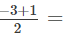-2/2 = -1
Question 2:  Find any five rational numbers less than 2.

We can write: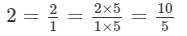Integers less than 10 are 0, 1, 2, 3, 4, 5 ... 9.

Hence, five rational numbers less than 2 are 0/5, 1/5, 2/5, 3/5,and 4/5 .
Question 3: Find two rational numbers between −2/9 and 5/9.-29 and 59.

Since both the fractions (−2/9 and 5/9) have the same denominator, the integers between the numerators(−2 and 5) are −1, 0, 1, 2, 3, 4.Hence, two rational numbers between −2/9 and 5/9 are 0/9 or 0 and 1/9.
Question 4: Find two rational numbers between 1/5 and 1/2.15 and 12
Answer 4: Rational number between1/5 and1/2 =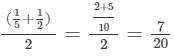Rational number between 1/5 and7/20 =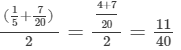Therefore, two rational numbers between 1/5 and 1/2 are 7/20 and 11/40.
Question 5: Find ten rational numbers between 1/4 and 1/2.14 and 12.
Answer 5: The L.C.M of the denominators (2 and 4) is 4.

So, we can write 1/4 as it is.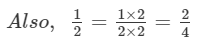As the integers between the numerators 1 and 2 of both the fractions are not sufficient, we will multiply the fractions by 20.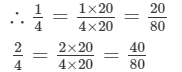Between 20 and 40, there are 19 integers. They are 21, 22, 23, 24, 25, 26, 27....39, 40.

Thus, 21/40,22/40,23/40,24/40,25/40,...................38/40 and 39/40 are the fractions.We can take any 10 of these.

Question 6: Find ten rational numbers between -2/5 and1/2. and 12.

L.C.M of the denominators (2 and  5) is 10.

We can write: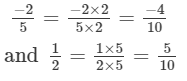Since the integers between the numerators (−4 and 5 ) of both the fractions are not sufficient, we will multiply the ractions are not sufficient, we will multiply the fractions by 2.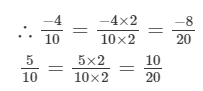There are 17 integers between −8 and 10, which are −7,−6,−5,−4...................8, 9.These can be written as:

−7/20, −6/20, −5/20, −4/20, −3/20,................... 8/20 and 9/20

We can take any 10 of these.

Question 7: Find ten rational numbers Between 3/5 and 3/4.
Answer 7: The L.C.M of the denominators 5 and 4 of both the fractions is 20.We can write: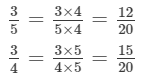Since the integers between the numerators 12 and 15 are not sufficient, we will multiply both the fractions by 5.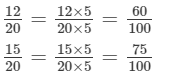There are 14 integers between 60 and 75. They are 61, 62, 63.......73 and 74. Therefore, 60/100,61/100,62/100..........73/100 and74/100 are the 14 fractions. We can take any 10 of these.

91 docs

,

,

,

,

,

,

,

,

,

,

,

,

,

,

,

,

,

,

,

,

,

,

,

,

,

,

,

;